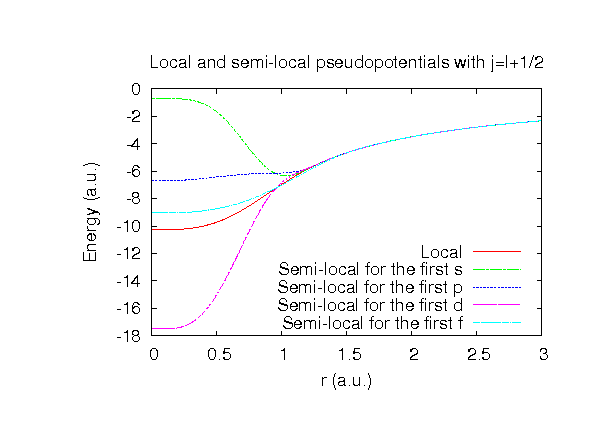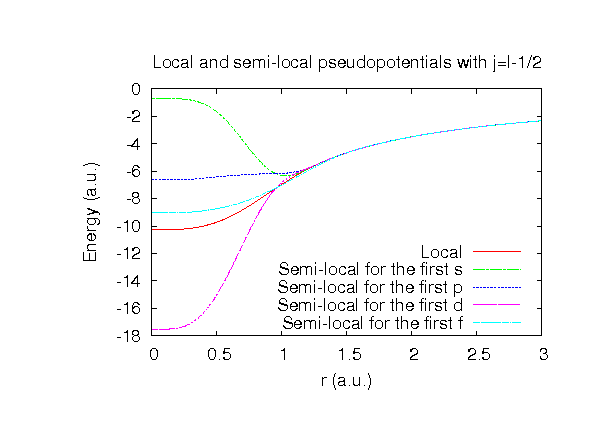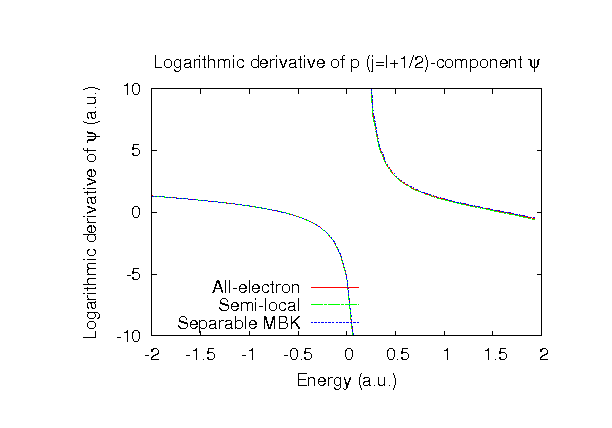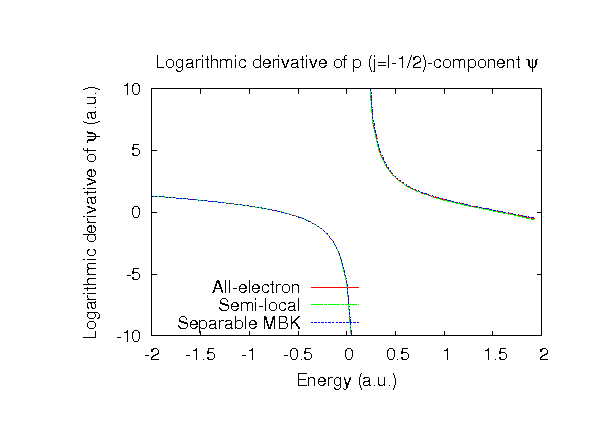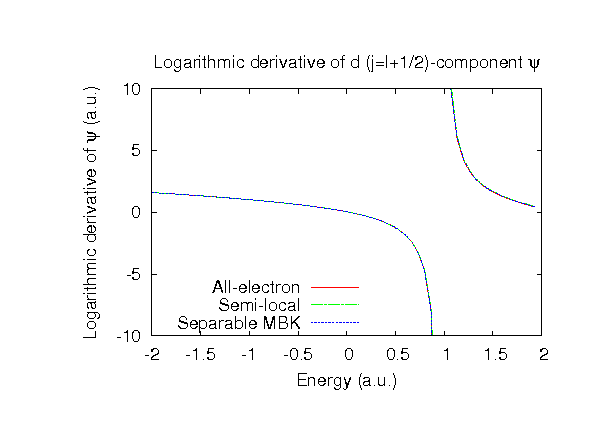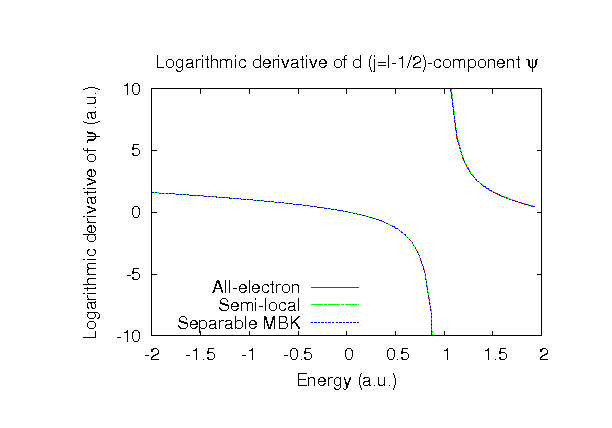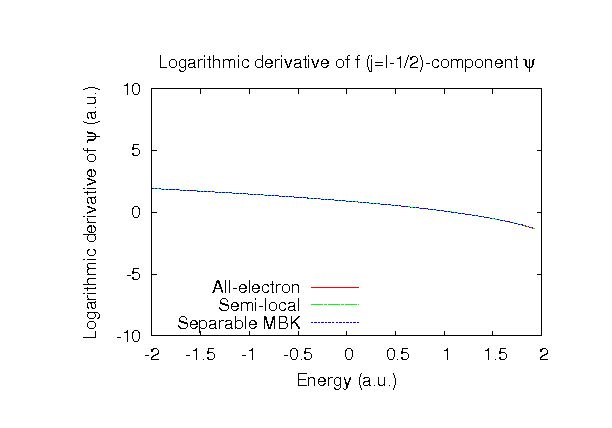### Fully relativistic pseudopotentials

Fully relativistic pseudopotentials generated by the MBK (PRB 47, 6728 (1993)) scheme within LDA (CA13) and GGA (PBE13) which contain a partial core correction and fully relativistic effects including spin-orbit coupling.

### Pseudo-atomic orbitals

The number below the symbol means a cutoff radius (a.u.) of the confinement potential. These file includes fifteen radial parts for each angular momentum quantum number l (=0,1,2,3,4). The basis functions were generated by variationally optimizing the corresponding primitive basis functions in the single atom, the dimer molecule, and the NaCl bulk. The input files used for the orbital optimization can be found at Cl_opt.dat , Cl2_opt.dat , and NaCl_opt.dat . Since Cl_CA13.vps and Cl_PBE13.vps include the 3s and 3p (7 electrons) as the valence states, the minimal basis set is Cl*.*-s1p1. Our recommendation for the choice of cutoff radius of basis functions is that Cl7.0.pao is enough for bulks, but Cl8.0.pao or Cl9.0.pao is preferable for molecular systems.

### Benchmark calculations by the PBE13 pseudopotential with the various basis functions

(1) Calculations of the total energy as a function of lattice constant of NaCl in the rock salt structure, where the total energy is plotted relative to the minimum energy for each case. a0 and B0 are the equilibrium lattice constant and bulk modulus obtained by fitting to the Murnaghan equation of state. The difference between Cl7.0-s2p2d1 and Cl7.0-s3p3d2 in the total energy at the minimum point is 0.00513 eV/atom, where Na9.0-s3p2d1 was used as basis functions for Na for both the cases. An input file used for the OpenMX calculations can be found at NaCl-EvsV.dat . For comparison the result by the Wien2k code is also shown, where the calculation was performed by default setting in the Ver. 9.1 of Wien2k except for the use of RMT x KMAX of 12. We note that the lattice constant of 5.681 Ang. that we calculated by Wien2k is much smaller than the value (5.714 Ang.) reported in PRB 75, 115131 (2007), although the same functional is used in both the Wien2k calculations.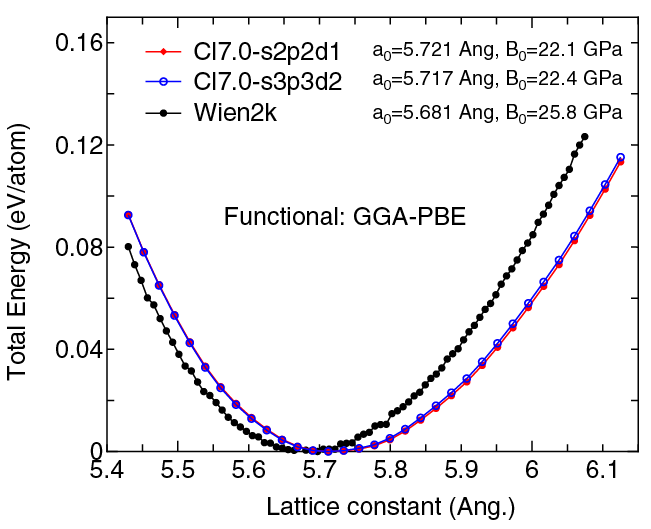(2) Calculations of the band dispersion of NaCl in the rock salt structure, where the non-spin polarized collinear calculation with the lattice constant of 5.63 Ang. was performed using Na_PBE.vps, Cl_PBE13.vps, Na9.0-s3p2d1, and Cl7.0-s2p2d1, and the origin of the energy is taken to be the top of the valence band. The input file used for the OpenMX calculations can be found at NaCl-Band.dat . For comparison the result by the Wien2k code is also shown, where the calculation was performed by default setting in the Ver. 9.1 of Wien2k except for the use of RMT x KMAX of 12.(3) Calculations of the total energy as a function of lattice constant of LiCl in the rock salt structure, where the total energy is plotted relative to the minimum energy for each case. a0 and B0 are the equilibrium lattice constant and bulk modulus obtained by fitting to the Murnaghan equation of state. The difference between Cl7.0-s2p2d1 and Cl7.0-s3p3d2 in the total energy at the minimum point is 0.0112 eV/atom, where Li8.0-s3p2d1 was used as basis functions for Li for both the cases. An input file used for the OpenMX calculations can be found at LiCl-EvsV.dat . For comparison the result by the Wien2k code is also shown, where the calculation was performed by default setting in the Ver. 9.1 of Wien2k except for the use of RMT x KMAX of 12.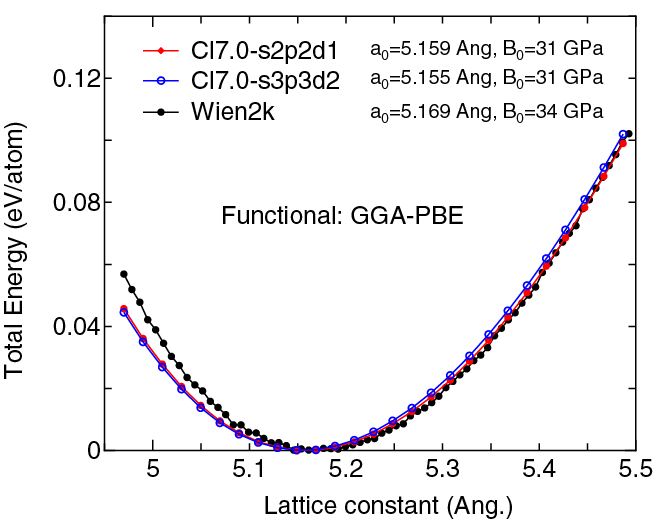(4) Calculations of the band dispersion of LiCl in the rock salt structure, where the non-spin polarized collinear calculation with the lattice constant of 5.106 Ang. was performed using Li_PBE.vps, Cl_PBE13.vps, Li8.0-s3p2d1, and Cl7.0-s2p2d1, and the origin of the energy is taken to be the top of the valence band. The input file used for the OpenMX calculations can be found at LiCl-Band.dat . For comparison the result by the Wien2k code is also shown, where the calculation was performed by default setting in the Ver. 9.1 of Wien2k except for the use of RMT x KMAX of 12.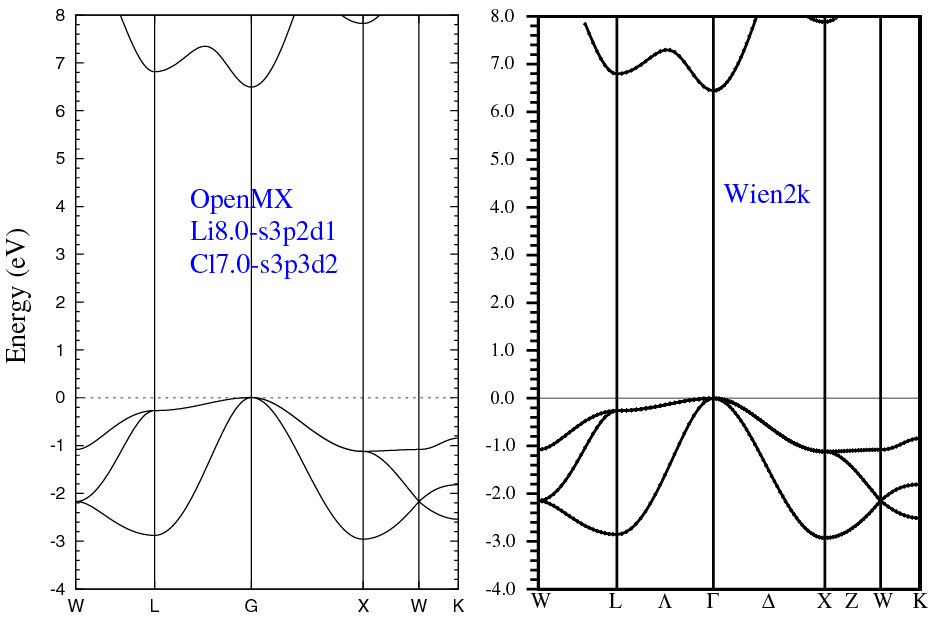(5) Calculations of the Cl2 molecule with S=1, where Cl_PBE13.vps and Cl9.0-s3p3d2 or Cl9.0-s4p4d4f3 were used. The input files used for the OpenMX calculations can be found at Cl2.dat , Cl.dat , and Cl_CP.dat .

 Equilibrium bond length (Ang.) Atomization energy (eV) Atomization energy (couterpoise corrected) (eV) Cl9.0-s3p3d2 2.053 2.72 2.72 Cl9.0-s4p4d4f3 2.021 2.96 2.96 Other calc. 2.018 a 2.86 a Expt. 1.988 b 2.51 b

a F. Tran et al., Phys. Rev. B 75, 115131 (2007).
b Taken from Ref. (a)

### Supplementary information for the GGA (PBE13) pseudopotential